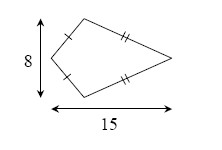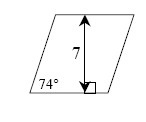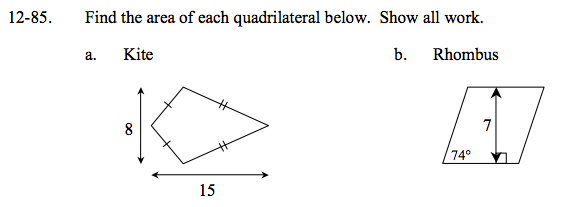### Home > GC > Chapter 12 > Lesson 12.2.4 > Problem12-85

12-85.
1. Find the area of each quadrilateral below. Show all work. Homework Help ✎

1. Kite2. Rhombus$\text{The formula for the area of a kite is} \frac{d1 \cdot d2}{2}.$

$\frac{(8)(15)}{15}=\frac{120}{2}=60\;\text{un^2}$

Draw a right triangle inside the rhombus. Solve for the length of the slant using trig. Remember that all sides of the rhombus are the same length.